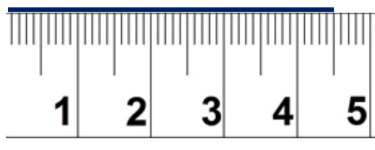# Math Worksheets Land

Math Worksheets For All Ages

# Math Worksheets Land

Math Worksheets For All Ages

When we get into our first real measurement unit, we begin to teach students how to measure length with a standard ruler. The first skill I start students off with is simply learning how to line a ruler up with what we are trying to measure. While this seems like a no-brainer, many students have difficulty with it. Before you help them make sense of the measure itself, make certain they can line it up properly. When that is mastered, I begin to teach them about the scale on a ruler. Regardless of which measurement system you are focused on using, I highly recommend that you start with a metric ruler. The base ten system rolls right along with this form of measure. I find that after a few days of practice with metrics, they are ready to move on to other systems, if required. These worksheets and lessons will help students learn this skill and how to apply it many different types of objects.

### Aligned Standard: Grade 3 Measurement - 3.MD.4

• Answer Keys - These are for all the unlocked materials above.

### Homework Sheets

We use U.S. Standard and metric length measurement units here.

• Homework 1- If we measure the length by the ruler we can do this by counting the numbers on the ruler. The first measurement (a) starts at 1 and continue to 6.
• Homework 2- Centimeters are the long marks on the ruler and denoted by the numbers. One millimeter is equal to one-thousandth of a meter (0.039 in.).
• Homework 3- If we measure the length by the ruler we can do this by counting the numbers on the ruler. The first measurement (a) starts at 1 and continue to 8.

### Practice Worksheets

Note that the measures are not lined up at the zero column. This is how students are assessed.

• Practice 1- Write the measures of each ruler below using the indicated units.
• Practice 2- Write the measures that you read as you go through this worksheet.
• Practice 3- Inches are units of linear measure that is equal to one-twelfth of a foot.

### Math Skill Quizzes

Use the units that cited at each stage of the quiz.

• Quiz 1 - Fire away! This is fun because each problem has you measure in metric and Imperial units.
• Quiz 2 - Do expect any less than that first quiz? I hope not.
• Quiz 3 - Why would you want to find these values in any less time?

### How to Read a Ruler

When a kid is learning about length and how to determine the value of it, a ruler is the first measurement tool they are introduced to. It is the most basic tool in measurement, mathematics, and geometry. It usually provides length readings in inches, centimeters, and millimeters. This all depends on the type of ruler that you have available.

A metric ruler reads measurements in centimeters and millimeters. The smaller ticks on represent millimeter readings, and the longer ticks represent centimeter. There are 9 small ticks in one centimeter. Ten millimeters make up one centimeter. You can see a metric ruler below. As you can see each number listed at the bottom represents a centimeter (the large tick). The smaller ticks are all millimeters. If we were to measure the length of the blue line above the ruler. We can see that it has past the 4-centimeter mark, but not the 5-centimeter mark. So, the first reading is 4 centimeters. We then need to determine how many small ticks (millimeters) this line measures. We can see that it covers 5 millimeters. The length of this line is 4 centimeters 5 millimeters or 4.5 centimeters.The line of sight is an important factor when it comes to accurately reading your measurements on a ruler. The line of sight must be perpendicular to the ruler. Deviation in the angle between the line of sight and the placement of the tool will pave the way for inaccurate measurement reading.

Example: Make sure that one end of the object you are measuring is coinciding the zero mark on the ruler. Keep your eye just above the ruler. Use the tip of the pencil to check the tick the end of the object is coinciding with. These worksheets will help you learn to master these skills.

Unlock all the answers, worksheets, homework, tests and more!
Save Tons of Time! Make My Life Easier Now

## Thanks and Don't Forget To Tell Your Friends!

I would appreciate everyone letting me know if you find any errors. I'm getting a little older these days and my eyes are going. Please contact me, to let me know. I'll fix it ASAP.